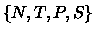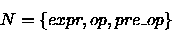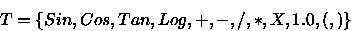Next: Grammatical Evolution Up: Introduction Previous: Introduction

## Backus Naur Form

Backus Naur Form (BNF) is a notation for expressing the grammar of a language in the form of production rules. BNF grammars consist of terminals, which are items that can appear in the language, i.e +, - etc. and non-terminals, which can be expanded into one or more terminals and non-terminals. A grammar can be represented by the tuple,, where N is the set of non-terminals, T the set of terminals, P a set of production rules that maps the elements of N to T, and S is a start symbol which is a member of N. For example, below is the BNF used for this problem, whereS = <expr>

And P can be represented as:

(1) <expr> ::= <expr> <op> <expr>     (A)
| ( <expr> <op> <expr> ) (B)
| <pre-op> ( <expr> )    (C)
| <var>                  (D)

(2) <op> ::= + (A)
| - (B)
| / (C)
| * (D)

(3) <pre-op> ::= Sin

(4) <var> ::= X   (A)
| 1.0 (B)


Unlike a Koza-style approach, there is no distinction made at this stage between what he describes as functions (operators in this sense) and terminals (variables in this example), however, this distinction is more of an implementation detail than a design issue.

Whigham [Whigham 96] also noted the possible confusion with this terminology and used the terms GPFunctions and GPTerminals for clarity. We will also adopt this approach, and use the term terminals with its usual meaning in grammars.

For the above BNF, Table 1 summarizes the production rules and the number of choices associated with each.

Table 1: The number of choices available from each production rule.
 Rule no. Choices 1 4 2 4 3 1 4 2Next: Grammatical Evolution Up: Introduction Previous: Introduction
root
1998-10-02